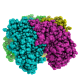# A periodic table of objects

… or a first step into Python objects.

Let us assume that for some large project we need an easy way to
translate atomic symbols into element names. The first thing that comes
to your mind is to use a dictionary. As this seems a bijective relation,
keys can be symbols and values names, or vice-versa. This is an
important decision as it fixes the structure of the dictionary. I
believe keys should be symbols. Let me give you a couple of reasons.
First, there is one element W, that has 2 names, Tungsten and
Wolfram. Second, you might image extending the dictionary to include
names in other languages. Therefore:

```
elementsDict=dict(H='hydrogen',
He='helium',
Li='lithium' ) # And so on...
```

Now your project has grown and you would like to also keep the atomic
number of an element. Two simple solutions are creating a second
dictionary (duplicating work is not nice and error prone) or keeping a
tuple (or a list) for the values, such as:

```
elementsDict=dict(H=('hydrogen', 1),
He=('helium', 2),
Li=('lithium', 3) ) # And so on...
```

This makes accessing to the attributes of an element ugly, as you have
to remember the arbitrary order of the attribute in the tuple. Did I say
attributes? That sounds object oriented! In fact, it is easy to
visualize an element as something (an object!) with different
attributes such as its name, its atomic symbol, its atomic weight, etc.
These attributes are of different type and do not bear an ordered
relationship among themselves as a list assumes. So let’s make an
object:

```    class Element:
def __init__(self, name, atomicNumber):
self.name = name
self.atomicNumber = atomicNumber

elementsDict=dict(H=Element('hydrogen', 1),
He=Element('helium', 2),
Li=Element('lithium', 3) )
```

Let’s try it:

```
for symbol in elementsDict:
print("The atomic number of {} is {}".format(elementsDict[symbol].name,
elementsDict[symbol].atomicNumber))
```

The atomic number of hydrogen is 1
The atomic number of lithium is 3
The atomic number of helium is 2

At this point, you might think it would be useful for the element object
to also contain its symbol. That’s easy, because you can add attributes
to an object dynamically:

```
for symbol in elementsDict:
elementsDict[symbol].symbol = symbol

print("{} name is {}".format(elementsDict['H'].symbol,
elementsDict['H'].name))
```

H name is hydrogen

### The other way around

For the sake of completeness, imagine you have created a list of
elements with a class that contains the atomic symbol, and you then want
to create a dictionary from that list. I find this more clear as the
`__init__` method then contains all the attributes. Here is how you
can do it:

```
class Element2:
def __init__(self, symbol, name, atomicNumber):
self.symbol = symbol
self.name = name
self.atomicNumber = atomicNumber

elements2List =[Element2('H', 'hydrogen', 1),
Element2('He', 'helium', 2),
Element2('Li', 'lithium', 3) ]
# Then create a dictionary with a comprehension:
elements2Dict = {element.symbol: element for element in elements2List}
```

Obviously it works as before:

```
for symbol in elements2Dict:
print("The atomic number of {} is {}".format(elements2Dict[symbol].name,
elements2Dict[symbol].atomicNumber))
```

The atomic number of hydrogen is 1
The atomic number of lithium is 3
The atomic number of helium is 2